# AP Physics 2 Practice Test 11

### Test Information10 questions18 minutes

1.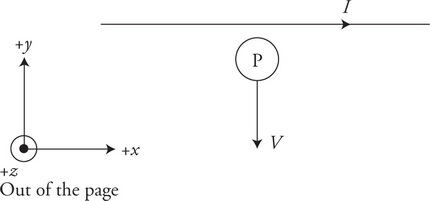A proton with a velocity v is moving directly away from a wire carrying a current I directed to the right in the +x direction as shown in the figure. The proton will experience a force in which direction?

2.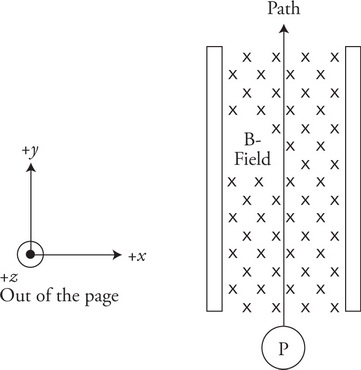A magnetic field directed into the page in the −z direction is placed between the plates of a charged capacitor as shown in the figure. The magnetic and electric fields are adjusted so that a particle of charge +1 e moving at a velocity of v will pass straight through the fields in the +y direction. Which of the following changes will cause the particle to deflect to the left as it passes through the fields?

3.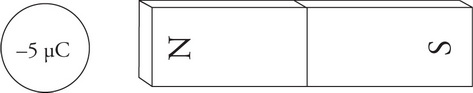A stationary charge is placed a small distance from an iron bar magnet as shown in the figure. Which of the following correctly indicates the cause and direction of the force on the charge?

4.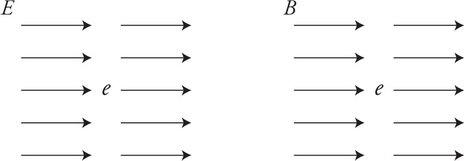An electron e is placed in an electric field and a second electron is placed in a magnetic field as shown in the figure. Both are released from rest at the same time. How do the forces on the charges compare?

5.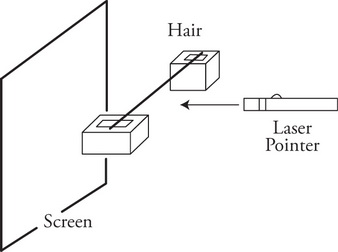A technician sets up a red laser pointer and directs light at a strand of hair suspended between two blocks in such a way that the light falls on a screen behind the hair as shown in the figure. This produces a pattern of alternating light and dark fringes on the screen. The technician makes one change to the setup and repeats the procedure. This change produces a similar pattern, but the light and dark fringes are spaced farther apart. Which of the following could account for this different pattern?

6.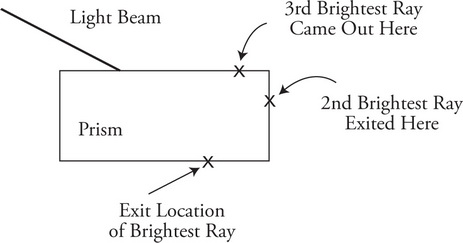Your lab group has been asked to determine the index of refraction of a plastic rectangular prism. Your partner places the prism on a sheet of paper, traces its shape, shines a beam of light through the prism, and traces the incoming ray. The light exits the prism at multiple locations. Your partner marks the exit locations of the three brightest rays on the side of the prism as shown in the figure. Is this enough information to determine the index of refraction of the prism and why?

7. A very slow-moving positron interacts with a stationary electron. Which of the following statements correctly describes a possible outcome of this reaction and why it would occur?

8.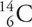decays into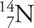and an electron. The half-life of carbon-14 is 5730 years. Which of the following statements is correct?

9.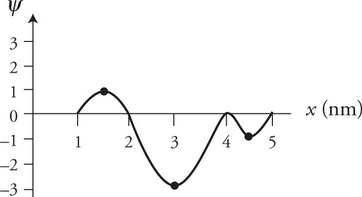The figure shows the wave functions ψ (x) of a particle moving along the x-axis. Which of the following statements correctly interprets this graph?

10.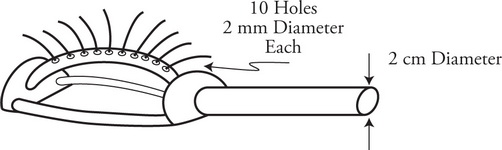A 2-cm diameter hose leads to a lawn sprinkler with ten 2-mm exit holes as shown in the figure. The velocity of the water in the hose is v1. What is the velocity of the water exiting the holes?